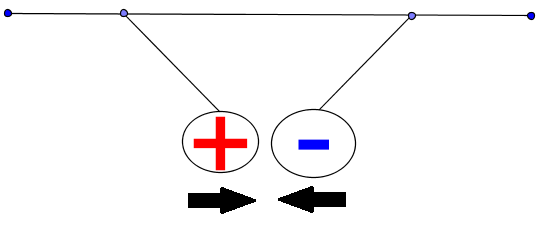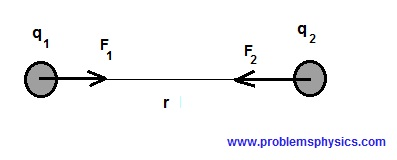#Physics Problems with Solutions# Electric Forces and Coulomb's Law

Tutorials on electric forces with examples and explanations.

## Electric Forces

Electric charges of the same sign repel each other and charges of opposite signs attract each other.

Charges of opposite signsBoth charges are positiveBoth charges are negative## Coulomb's Law

The forces on two charges of opposite signs for example, separated by a distance r are quantified by Coulomb's law as follows:$|F_1| = |F_2| = k \dfrac{|q_1 q_2|}{r^2}$
where $|F_1|$ and $|F_2|$ are the magnitudes of forces $F_1$ and $F_2$, $r$ is the distance separating the two charges $q_1$ and $q_2$ and $k$ is a constant.

## More Than Two Charges

In the example below, there are more than two charges: $q_1$ positive and $q_2$ and q3 negative. There are two forces acting on q1 and the resultant force is the sum of the forces $F_1$ + $F_3$ (addition of vectors since the forces are represented by vectors)